# Inter Maths Solutions for Exercise 10(b) Properties of Triangles

Inter Maths 1A text book Exercise 10(b) Properties of Triangles solutions are given.

These solutions are very easy to understand.

Please study the text book lesson Properties of Triangles very well.

Observe the example problems and solutions given in the text book. Try them well.

Observe the given solutions and try them in your own method.

You can also see

Inter Maths 1A textbook solutions

Inter Maths 1B textbook solutions

Inter Maths IIA textbook solutions

Inter Maths IIB textbook solutions

Properties of Triangles

Exercise 10(a)

Exercisr 10(b)

M

## Maths 1A Inter Solutions for Exercise 10(b) Properties of Triangles

Chapter – 10

Properties of Triangles

Exercise 10(b)## Maths Solutions for 1A Properties of Triangles Exercise 10(b)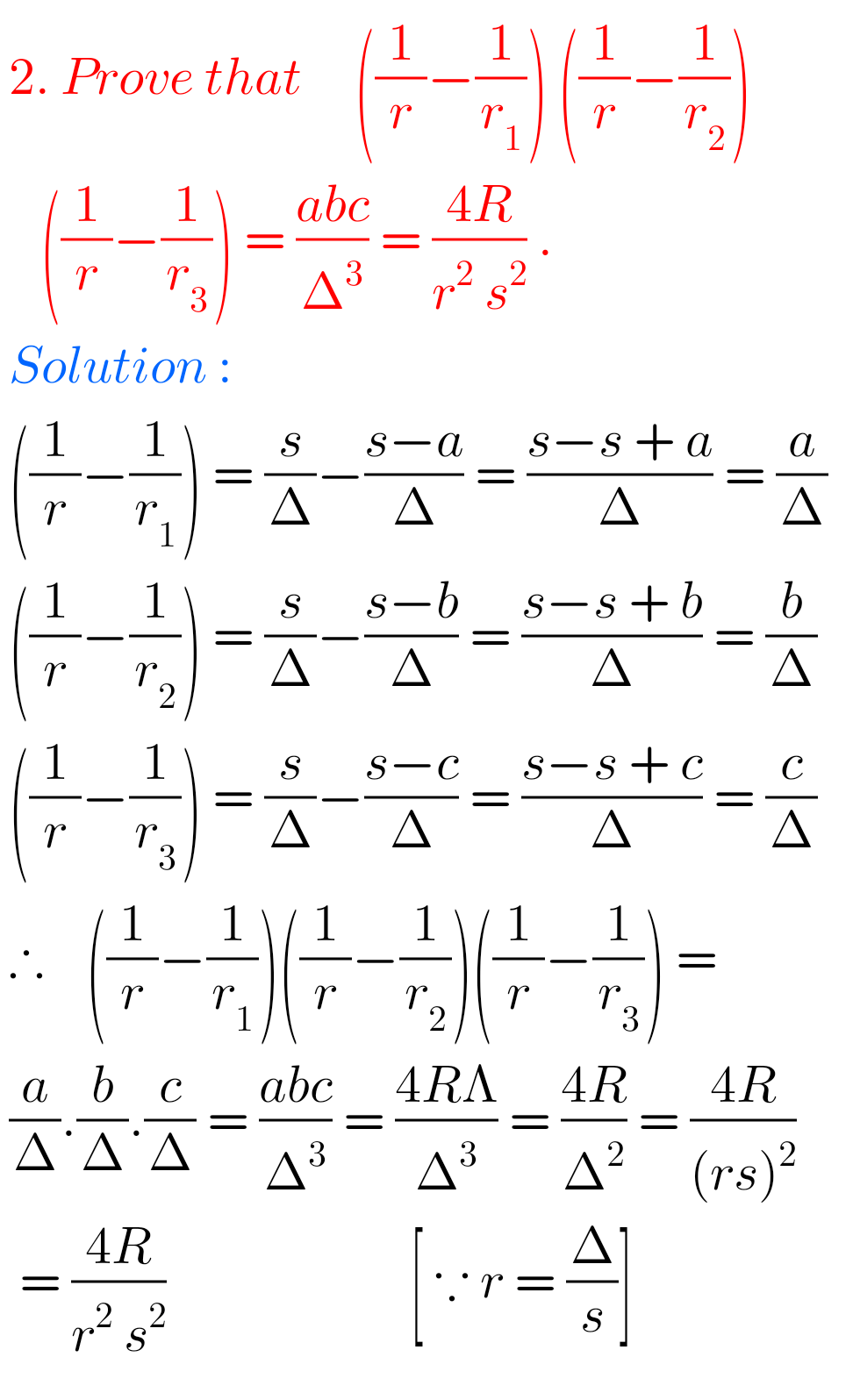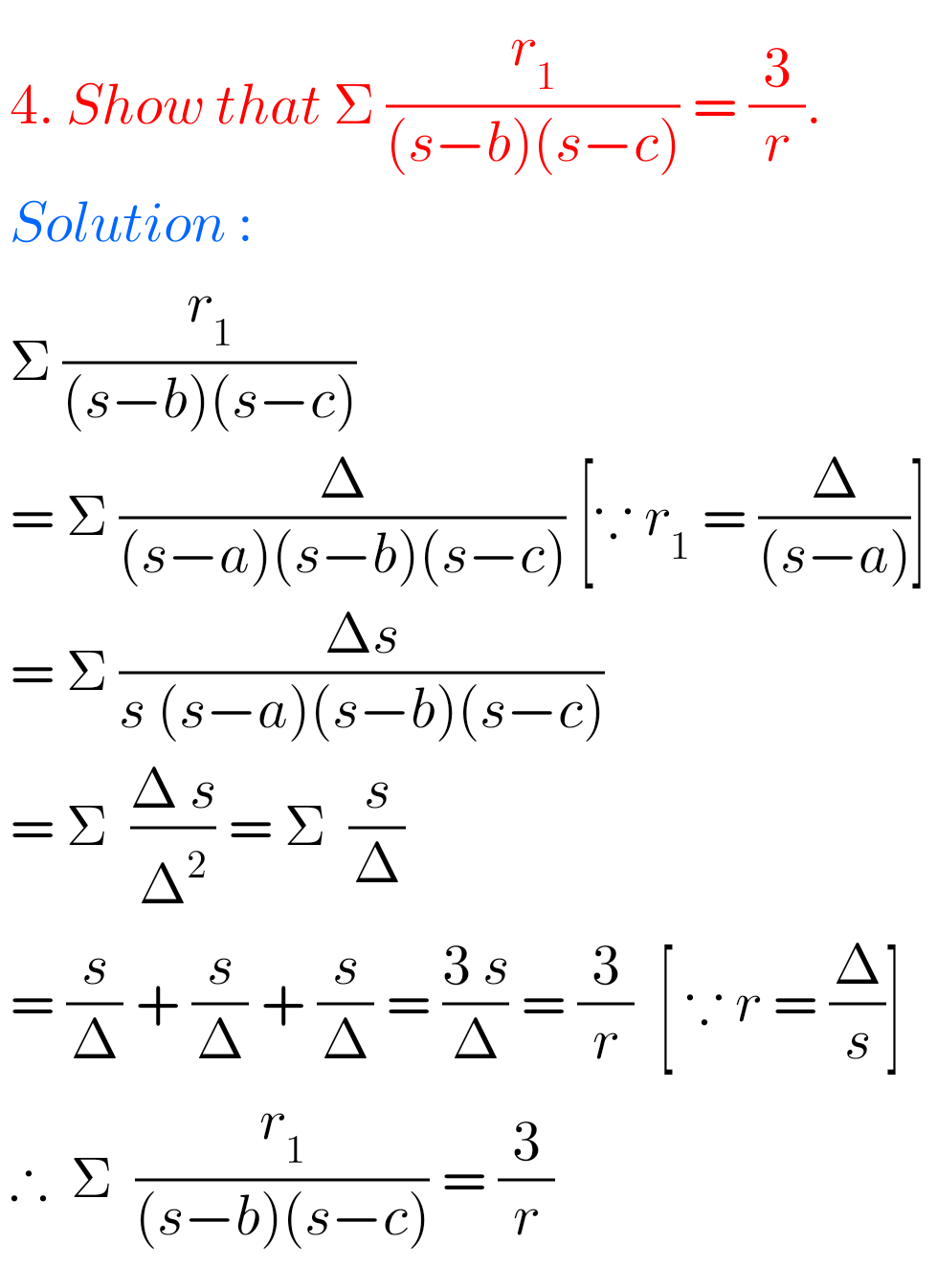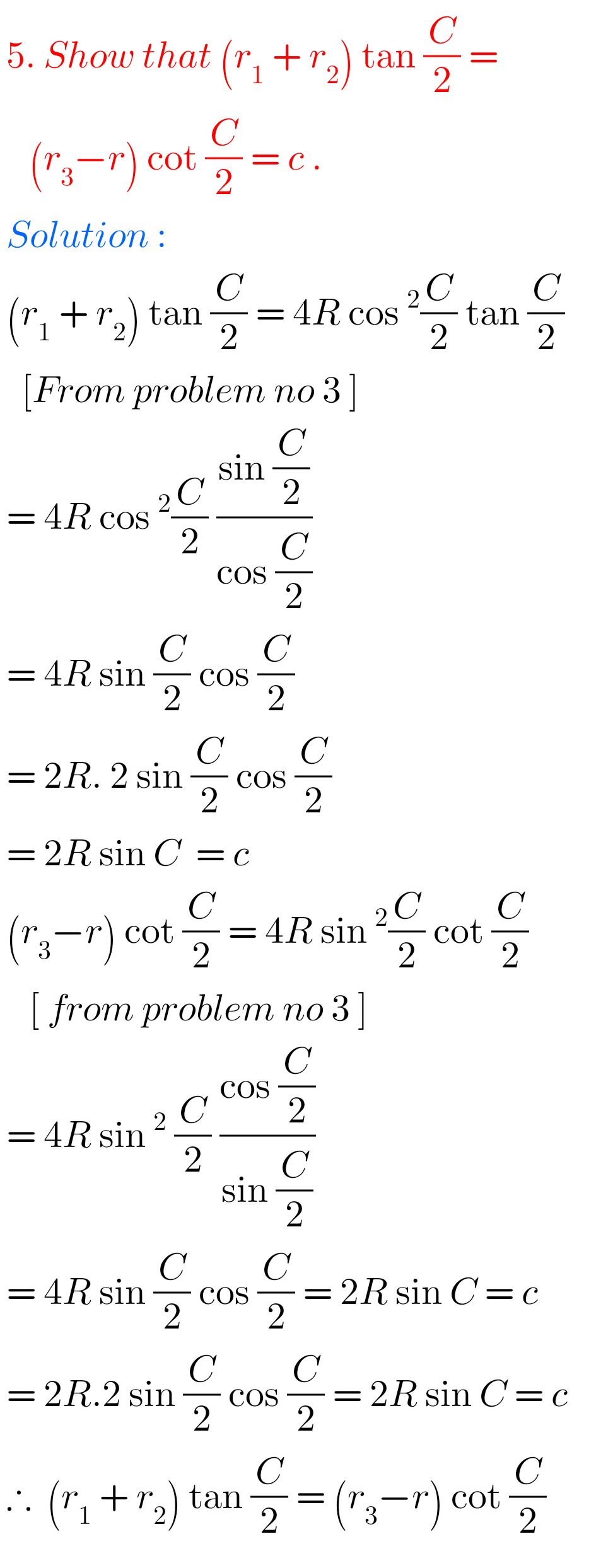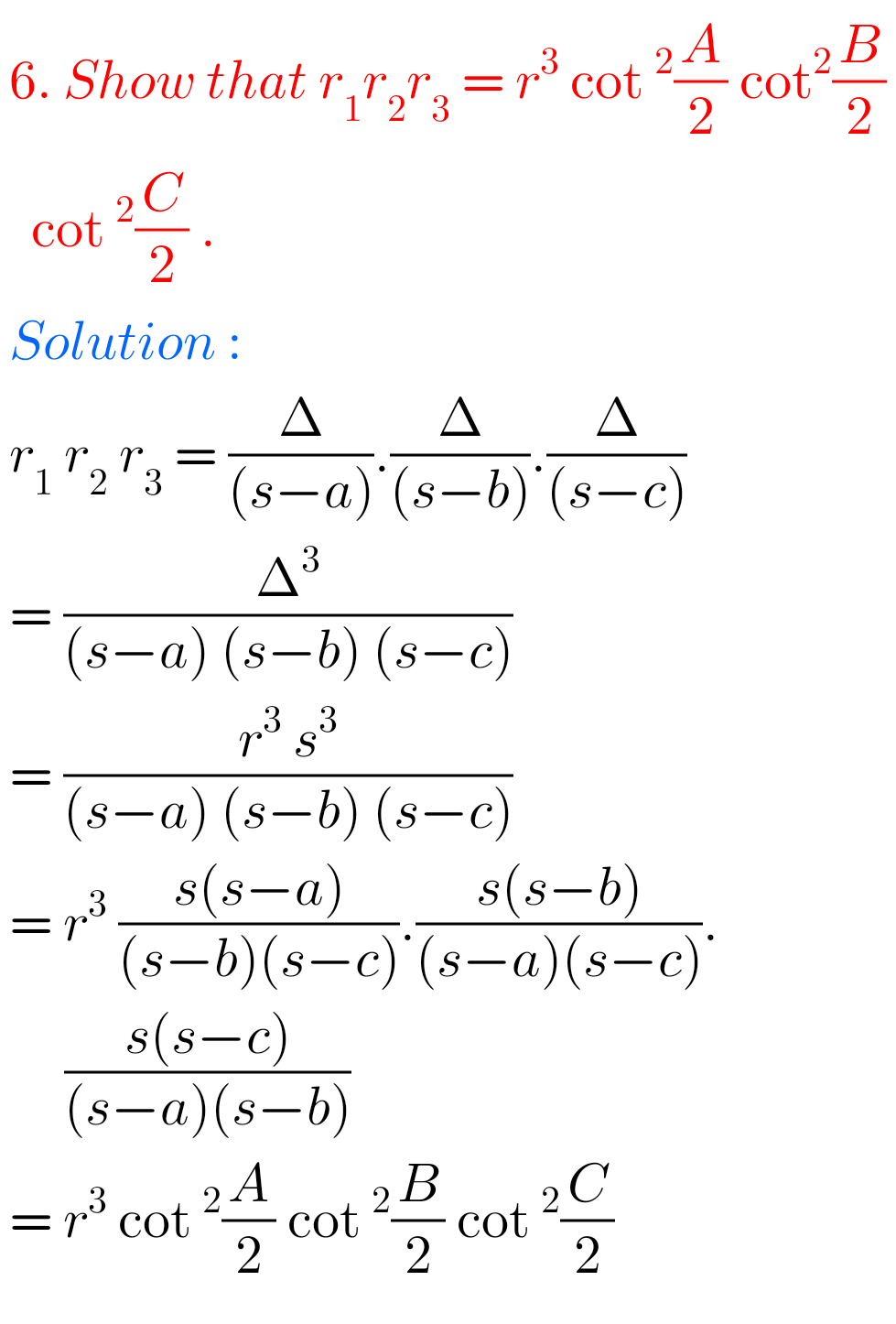## Properties of Triangles Exercise 10(b) Solutions Inter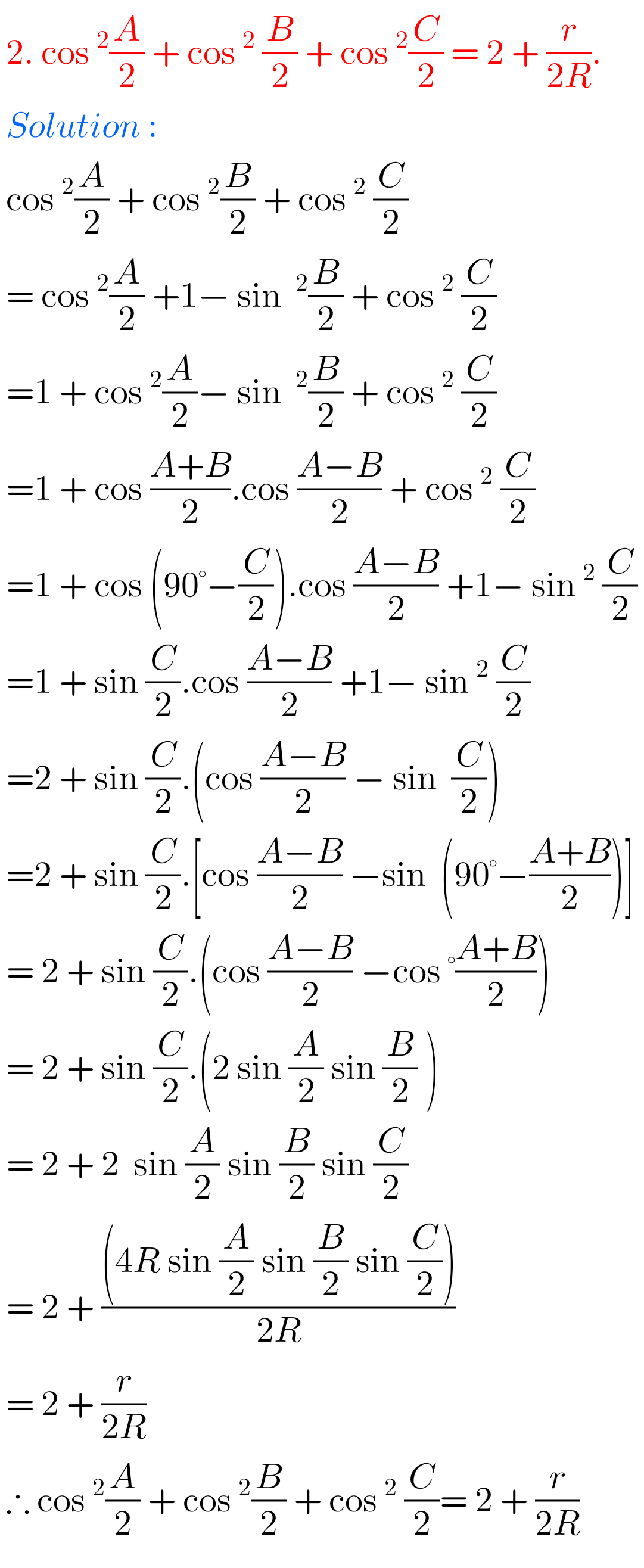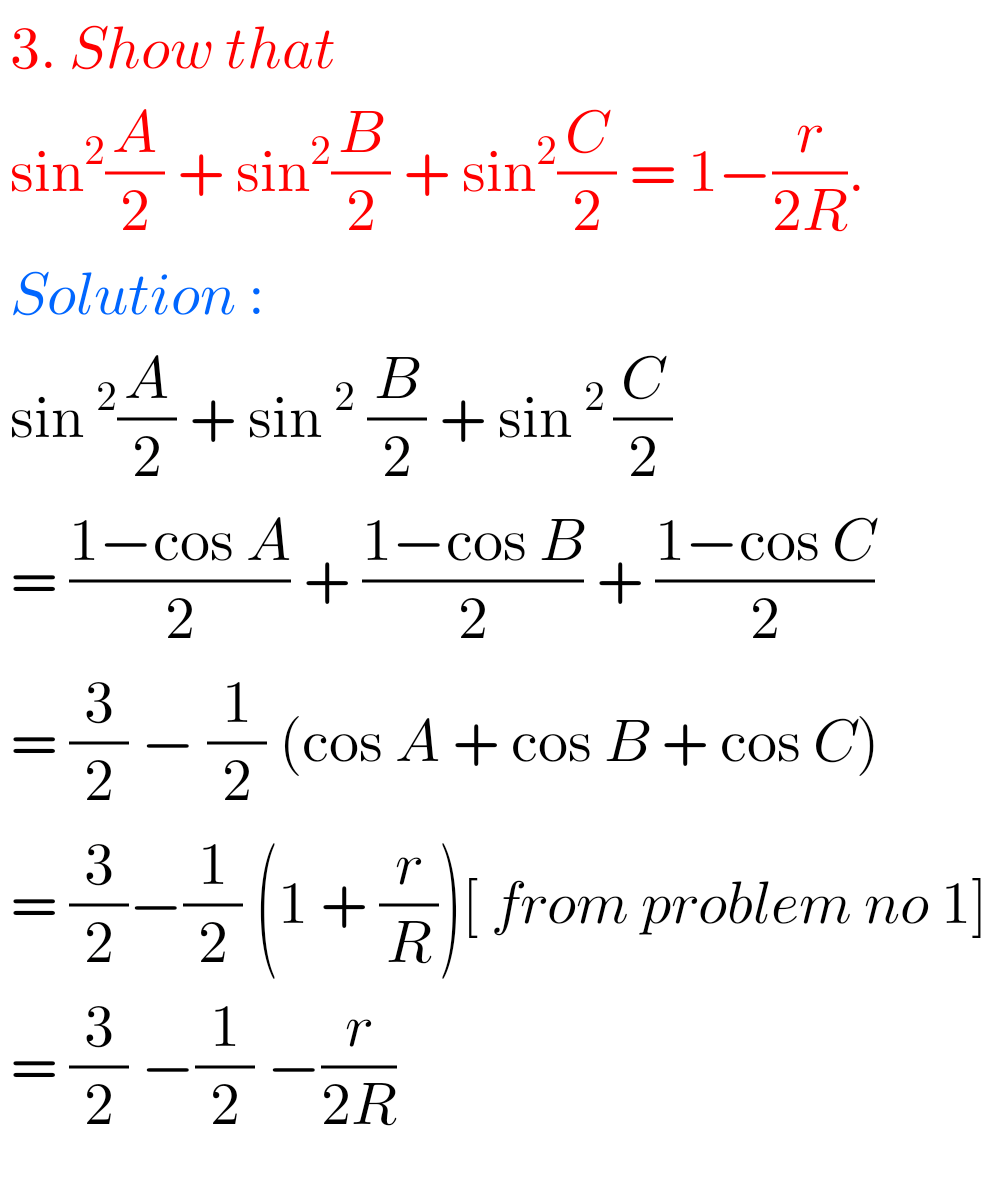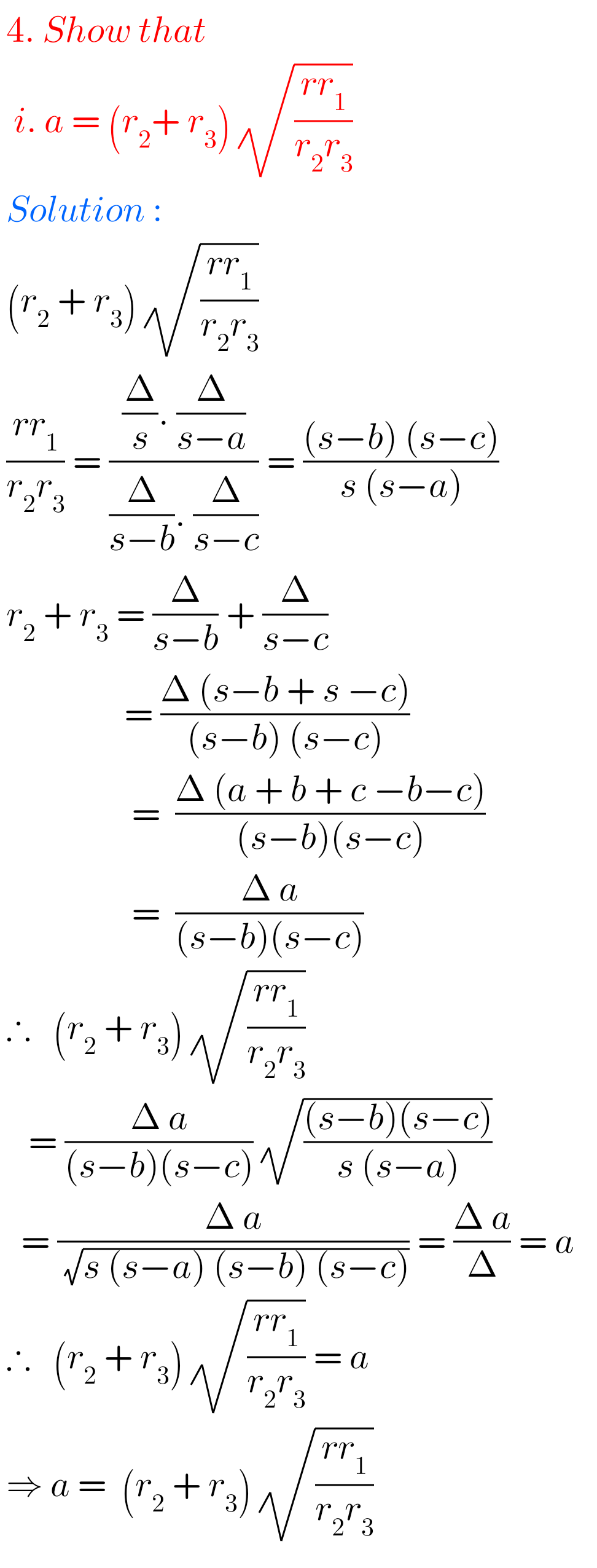Note : Observe the solutions and try them in your own method.

Some moreYou can also see

SSC Maths text book Solutions class 10

You can see the solutions for junior inter maths 1A for examination purpose

2. Matrices